Search IntMath
Close

450+ Math Lessons written by Math Professors and Teachers

5 Million+ Students Helped Each Year

1200+ Articles Written by Math Educators and Enthusiasts

Simplifying and Teaching Math for Over 23 Years

# The equal sign - more trouble than it’s worth?

By Murray Bourne, 25 Aug 2010

What to do with the equal sign?

## A common problem

7 + 3 = 10 + 3 = 13 − 8 = 5

Now, each of the operations is done correctly, but the whole thing is actually not correct since the first expressions (7 + 3 and 10 + 3) don't equal the last expression (13 − 8) or the final number.

This is called a "running equals" and it is quite wrong.

In the article Students' understanding of equal sign, we learn that 70% of middle grade students in the US had misconceptions about the equals sign (Research by Robert M. and Mary Capraro of Texas A&M University.)

As an example, they cite the following question:

4 + 3 + 2 = ( ) + 2

Many students will incorrectly add everything on the left, put that in brackets, then proceed to add the final 2.

4 + 3 + 2 = (9) + 2 = 11

The question really requires the following:

4 + 3 + 2 = (7) + 2 = 9

Why do students make such mistakes?

## Background

One of the first things we learn in math is the equal sign. We count 4 blocks and add 2 blocks and get 6 blocks. Some (well-meaning) person tells us we can write this as follows:

4 + 2 = 6

Did you realize that the equals sign is a relatively recent invention, and that mathematicians did just fine without it for thousands of years?

The equals sign, "=" was invented by a man from Wales, Robert Recorde in the mid-16th century. He used equal-length parallel lines because "nothing could be more equal".

Before the invention of the "=" symbol, mathematicians employed useful and expressive words to indicate what was going on. So when adding, they used "gives" or "yields". Other early symbols for equals included "æ" (from the Latin "aequalis") and 2 vertical parallel lines, ||.

Over the years, several different meanings have evolved for the "=" sign, and this can increase student confusion.

#### Meaning #1 - result of performing an operation

In the 4 + 2 = 6 example, "get 6 blocks" in my English sentence is written as "= 6" when translated into a statement using symbols only.

#### Meaning #2 - decomposition

What if we flip the above statement and write it as follows?

6 = 4 + 2

Now the "=" sign doesn't represent the same concept as our "=" sign in the earlier 4 + 2 = 6 example.

Now it means we start with 6 and we have decomposed it into 4 + 2. But we could also decompose 6 in any of the following ways:

6 = 3 + 3
6 = 2 + 4
6 = 5 + 1
6 = 0 + 6

This time we have an infinite range of answers. Clearly "=" here is used differently than in the 4 + 2 = 6 example, where we had one possible answer (assuming we are only talking about real numbers, of course), while the second set of examples starts with a single number and suggests a very open-ended set of solutions.

#### Meaning #3 - equivalent value of 2 operations

Now, let's look at another case.

5 + 9 = 7 × 2

Here the "=" sign means something different again. Now we need to do something to the expression on the left (add them) and do something different with different numbers on the right (multiply them) and after all that, we can conclude that indeed both sides have the same value.

#### Meaning #4 - equivalence in sets

In set theory, "equal" is not the same as "equivalent".

For example, the set {1,2,3} is equivalent to the set {a,b,c} since they both have the same number of elements (the same cardinality), but they are not equal since they don't contain exactly the same elements.

Also, we can write {1, 2, 3} = {2, 1, 3} (they are equal) since the elements in the set are exactly the same, but the order doesn't matter.

#### Meaning #5 - equivalence in equations

We can have equivalent equations. For example, the following 2 equations are equivalent since they have the same solution set:

x + 2 = 7
3x = 15

Both equations give us x = 5. We can't say we have "equal equations", but we do use the term "equivalent".

#### Meaning #6 - equivalence in ratios

Two fractions can be equivalent:

class="indent"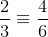They look different but they have the same value.

Notice I used the "3 horizontal lines" symbol (≡) for "equivalent". We can also say "equal" in this example.

#### Meaning #7 - as a limit

This next one causes a lot of debate. Start with 0.9. Then, take the larger number 0.99. We have moved closer to 1, correct?

Now, keep going. 0.99999... Clearly, if we go (infinitely) far enough, we will end up with this result:

0.999... = 1

This employs a very different concept to the first 3 meanings of "=" given above. Is it exactly equal to 1? Don't we need a "limit" statement of some kind?

Here's another one. Being irrational, pi cannot exactly "equal" some number. Or can it?

We can show that pi has the following value: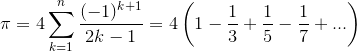Should we write "=" or "≈" (approximately equal)?

#### Meaning #8 - use in proofs

Here's another place where many students come unstuck - proving trigonometry identities. Say the question is:

Prove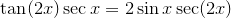Many students attempt a solution something like this: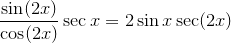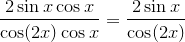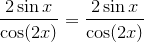Now essentially the steps are correct, but it is difficult to follow the logic. Should we go left-to-right, or top to bottom, or a mix of the two?

What the student has done is to work down the left side and right side in a parallel fashion until he got something that looked the same on both sides.

It's not the best proof, since there is no clear statement of what we start with and what we are doing in each step.

## Some possible solutions for the "=" sign problem

Computer languages and computer algebra systems use different symbols for the different meanings of the equals sign.

This might be a good solution for mathematics.

In PHP computer language, for example (which IntMath and squareCircleZ uses), "=" is used to assign the value of a variable, while "==" is used to check for equality.

So for example, the following statement means variable "a" will have value 5 from this point on in the program.

a = 5

If we need to test to see if some condition is true, for example whether variable "b" has value 3, we would write:

if ( b == 3 ) {
[do something...]
}

In the computer algebra system Mathematica, the "==" sign is used for solving equations, as follows:

Input: Solve[2x == 6, x] Output: {x->3}

Note the "->" symbol for equality in the output.

For comparison, the rival software Mathcad has 4 different equal signs:

Assignment (:=) To assign a value to a variable, you need to use the "colon equal" notation which looks like this:

a := 5

Display a value (=) To display the value of variable "b" in Mathcad, you just type "b=" and the value will appear:

b = 8

Symbolic equality (=) To evaluate a symbolic expression you use the "bold equals" symbol:

b + b = 2b

Global assignment (≡) This is the "identically equal to" symbol from mathematics. This is used when you want to fix a variable throughout a whole math worksheet, not letting it be changed by other variables:

c 8

This is a different sense from that used in mathematics, however. But as the identity symbol suggests a "strong equals", perhaps it is appropriate.

## Part of the problem?

This is a screen shot from a video that extols the virtues of interactive white boards for math teaching.

The instructor's question is "Evaluate the equation". Can you really "evaluate an equation"?

You can solve an equation for some variable, or you can state that expressions are equal in an equation, but you can't evaluate an equation.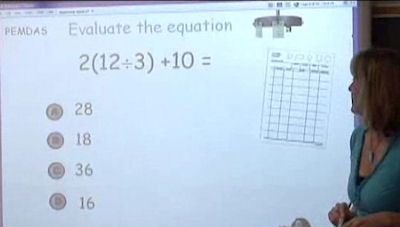Source: eSchoolNews

We need to be careful how we express mathematics questions since this is where misconceptions can arise.

## Summary

So why do students make mistakes with the equal sign? It's most probably because there are several nuances in meaning for the equal sign.

Perhaps we need to consider using a wider range of symbols for the different types of equals. One place to look is the notation used in computer languages or computer algebra systems.

### 28 Comments on “The equal sign - more trouble than it’s worth?”

1. gasstationwithoutpumps says:

While I agree that there is overloading of the equal sign, and misuse of it middle-school math, I'm not so sure I loke your taxonomy.

I see a few uses:

1) statement of equality. This makes a sentence that can be true or false. (4=2+2 is true, 5=3+1 is false). It is represented in many computer languages as "==". Your meanings 2, 3, 6, 7, and 8 are all this meaning.

Actually, the set equality is also, but I can understand wanting to separate it out, because of the confusion many students have about sets. Personally, I think the problem there is not with the meaning of "=" but with incomplete understanding of sets.

2) definition: Some symbol is defined to have a particular meaning. This is often represented as a triple-bar equivalence symbol or "=" with a subscript "def". This definitely deserves its own symbol.

3) assignment in computer languages (the ":=" you mentioned) which is generally not used in mathematics. It needs its own symbol, but probably should not be covered in math classes, as the semantics for assignment (involving change to the state of the world) are too messy for most math classes, and are often not covered formally in computer science classes except in junior and senior college electives.

4) the "running =" of calculators. This is the terrible thing that confuses American students about what "=" means in mathematics. I think the reason that American students have some much more problem with "=" is the overuse of calculators in early math classes. Personally, I think we should only allow RPN calculators in school, so that students never see an "=" on a calculator.

2. Aravind says:

I think "=" just implies that the RHS and LHS takes/has the same value.

3. shimonbentzvi says:

With all do respect, I think people who find this a problem have much too much free time.

4. Govindarajan says:

I also feel that the problem there is not with the meaning of “=” but with incomplete understanding of sets.

5. Alyson Vega says:

Like the first and second commenter, I agree that your breakdown of the = sign into many definitions is false. All of your subcategories are the same. 0.999... = 1 is not a limit nor an expression of a limit. They are equal in value. To avoid confusion in middle school students, elementary school educators need better math training. Do not teach students concepts which are false and students will not need to relearn them in high school.

6. Hypatia says:

In my experience, the remedial student's problems have usually stemmed both from mathematicians' ambiguous usage of the symbol “=” and also from the student's insufficient understanding of set theory built upon bivalent first-order logic (sentential calculus and quantification theory with identity) with membership.

I am an advocate of those Montessori educators who expose their pre-school age students to logic, set theory, and algebra before the introduction of arithmetic. In fact, I often recommend the use of their pre-school mathematics materials in tutoring remedial undergraduate students.

Traditionally, the Montessori language student is introduced to audible speech sounds before being introduced to letters as symbols for corresponding phonemes. He begins to write and to read words before he learns the audible names of the letters or their proper sequencing in the alphabet.

Analogously, the mathematics student works with sets of tangible objects before being introduced to numerals as symbols for corresponding numbers -- “0” for the null set, “1” for the set of all individuals, “2” for the set of all pairs, etc. He begins to write and to read numerals as names for these sets (i.e., as names for numbers) before he learns the audible (English) pronunciation of the names -- “zero”, “one”, “two”, etc. -- and before establishing the proper sequencing of the counting numbers (as he adopts “+” as the symbol for addition defined in terms of iteration of the successor function).

The student begins to construct, to read, and to determine the truth values of mathematical sentences using the binary predicate symbol “=”, arithmetic operator symbols, numerals, and the variables “x”, “y”, etc., just as he is beginning to construct, to read, and to determine the truth values of English sentences using the binary copula “is”, etc. The manipulable Montessori materials are color-coded such that the binary predicate “=” and the English copula “is” are imprinted on wooden blocks of one color, the variable “x” and the pronoun “it” on blocks of another color, etc. (The word “is” comes on blocks of different colors corresponding to its different usages in English -- identity, membership, existence, etc.)

The young student first learns to refer to “=” as the identity sign, but not as the equal sign, and to refer to properly constructed sentences using “=” as identifications or statements of identity, but not as equations or statements of equality. When reading expressions aloud, he learns to vocalize “=” as “is identical to” or simply as “is”, but never as “equals”. Only after the student has demonstrated a firm grasp of the concept underlying “=”, the concept of identity, and has expressed an interest in studying mathematical texts and related literature, is he warned that most mathematicians, some set theorists, and fewer logicians tend generally to refer to “=” as the equal sign and to identifications or statements of identity as equations or statements of equality.

Thus the student initially learns that the sum of the numbers one and two is (i.e., is identical to) the number three -- that 1+2=3. He also learns that the expression “the sum of one and two” is equivalent to the expression “three”, but that the two expressions are not identical -- that “1+2”≡“3”, but that “1+2”≠“3” because, although the semantically equivalent expressions name the same number, they differ in construction and appearance.

Additionally, the student already knows how to determine the truth value of “(x+2)×(x+1)=x²+3x+2” before he is ever introduced to the concept of place value and denary (base-ten) enumeration. Therefore, he needs no instruction before he can determine the truth value of “12×11=132”.

(Once, while visiting a Montessori school in Columbia, South Carolina, I had the experience of observing such a "pre-school" student. Immediately upon his initial introduction to the denary system, without the assistance of a calculator, for the first time in his life, and all in a single afternoon, he proceeded to correctly calculate sums, differences, products, and quotients, including long-division problems. When I left the school, he was in the process of calculating the square root of a number expressed as a five-digit numeral in base ten. It was later explained to me that, until recently, he had had great difficulty distinguishing between English letters such as “b” and “d” and between numerals such as “2” and “5” or even “6” and “9”. Consequently, he had been doing all of his work in mathematics using the quaternary system, since reversal or inversion of the numerals “0”, “1”, “2”, and “3”, was inconsequential to his work. However, I was also informed that he had never received any instruction in "long" calculations using the quaternary system either, but that he had had the benefit of having previously worked with polynomials as explained above.)

In light of my experiences, I applaud the general thrust of Murray's essay. However, I recommend a more radical approach, like that of the Montessori mathematics educators mentioned above, when first introducing mathematical concepts to young students. In my experience, I have never had to provide remedial tutoring to students who were predominantly the products of that radical approach. Furthermore, I have found the use of that approach very beneficial to high-school and undergraduate students who were in need of remediation.

BTW: gasstationwithoutpumps might be interested to note that the school I mentioned above supplied only RPN calculators to its youngest students. Also, the fact that, in their English language curriculum, they paid significant attention to the subjunctive mood might explain why their students seemed to have little difficulty grasping the semantics of “:=” when they begin learning a computer language during their pre-school years.

7. Alan Cooper says:

I agree with the first commenter above that the problem is exacerbated by the use of calculators, but long before that we had to deal with the inexcusable carelessness of some teachers, such as the author of the "evaluate the equation" example in your post.

I do think that several of your cases would be covered at once by making it clear that the statement A=B just means that "A" and "B" are two different ways of identifying the same object (and that operators aren't operations but just components of compound names - which, now that I think of it, is probably why I get so annoyed with the use of "plussing" and "timesing" for adding and multiplying).

But I also agree that the assignment or definition case and the algebraic identity case deserve special attention.

In these cases additional notation may be a convenient alternative to the use of appropriate surrounding language.

For example if we want to define a new variable we might say "b:=x+y" in place of "Let b denote a new variable satisfying b=x+y", and we might say "Is (x+1)(x-1)/x===x-1/x" instead of asking "Is (x+1)(x-1)/x=x-1/x true for all values of x for which one side is defined?"

8. Murray says:

Hi Alan and thanks for your input. Good to hear from you!

Now there's food for thought in this debate - "operators aren't operations".

9. gasstationwithoutpumps says:

Alan, please don't use := for "is defined to be". It took a lot of effort for computer scientists to accept a symbol for "is assigned the value". Reusing the same symbol for the completely different semantics of "is defined to be" would really mess things up. Use =_{def} for "is defined to be".

10. equal sign « Gas station without pumps says:

[...] — gasstationwithoutpumps @ 08:25 Tags: equals sign, math SquareCircleZ recently posted on the confusion that US students seem to have with multiple meanings of  the equal sign.  There has been considerable discussion [...]

11. Alan Cooper says:

Hi Murray,
That “operators aren’t operations” business was an afterthought and may not be the best way of putting it. (It certainly isn't something I would say to children for example.)

In fact, of course, it wouldn't be inconsistent to use "timesing" as the name of the operation that we call "multiplying". But then when we say "a times b" for "axb" it's really just a shorthand for "a timesed by b", and that may initially be as confusing to an English speaker as using the sentence "Jones marries Smith" as a noun phrase to represent the Jones-Smith couple.

So, although I never object directly to students who talk of "timesing", I do think that the standard language is less likely to encourage misinterpretation and so I always use it myself. And I regret that the reason some of my students deviate from it is not due to their own laziness but to that of their teachers.

Interestingly the same issue arises when students start learning about more general functions. The failure to distinguish the function f from the value f(x) is a source of much confusion and is encouraged by lazy references like "the function f(x)=x+1" rather than "the function f defined by f(x)=x+1".

cheers,
Alan

12. gasstationwithoutpumps says:

Distinguishing the function f from the expression f(x) is tricky, as most mathematicians are very sloppy about that notation (not just teachers). Teaching kids a programming language like Python in which functions are perfectly acceptable objects that can be bound to variables may or may not help them learn the distinction.

13. Alan Cooper says:

With regard to functions, one activity that I have found useful with a graphing tool is to have students define a function in terms of a variable other than x, by entering something like f(w)=2exp(-w^2)for example, and then to graph not just y=f(x), but also things like x=f(y), r=f(theta), f(x+y)=1 and so on.

And don't worry, I won't use := (or === ). I have just been too lazy to keep up with Murray on the on-line input of nice looking math notation, or even to type or find =_{def} and the three line identity sign.

14. Alan Cooper says:

At the risk of hogging space here I also wanted to comment on situation #7 - the case of limits.

I think it matters in this case whether students have been actually exposed to the limit concept. If their world consists just of finite decimal numbers then to tell them 0.999...=1 is not really fair, but once they have been exposed to limits and sums of geometric series, then I think it is ok to use the ... notation to represent the limit whenever it is "clear" from the context how the digits are to continue (even when it's a non-recurring case like 0.1010010001.... for example). And I certainly think that once we are working with real numbers we don't need an extra sign for equalities like the series expansion of pi.

But I also think that we do students a disservice any time we use (or let them get away with using) the equals sign for an approximation. So e=2.71828 is just plain wrong in my book. (I would accept e=2.718... though, but to say it right with the wiggly equals sign I'll have to go back and review those earlier postings on how to enter math notation)

15. Alyson Vega says:

Alan Cooper,

I agree with you that it would be incorrect to allow the shortening of e to 2.71828 and then use the identity/equal sign between them. In math text we write "is approximately using parallel squiggly lines.
When I teach fraction/decimal decimal concepts, inevitably there is a student who pursues the subject beyond the scope of the curriculum. The mention of limits is not necessary.

The teachable moment here is proofs. Is there a number between 0.999... and 1? If you divide both sides of the equation .999... = 1 by 3, have we maintained the equality?

My point is .9 repeating need not be discussed as a limit issue since .9 repeating does not approach 1. Only when we bring up the sum of the parts, do we need to discuss limits. 1 and .9 repeating are equivalent expressions for the same quantity.

The introduction of limits may cause confusion about equivalency as students can have difficulty absorbing the notion that sums approaching a number means the limit equals a number.

Bottom line in my mind is = sign says "is", "equals", "is equal to", "is defined as" for this particular problem are the same and mesrely a semantic discussion.

The problem arises in elementary education when students think it means "the simplification of the expression directly to its left." My proposal introduce algebraic terminology earlier.
Expressions: 3+2, 40÷8, 6-1 are all expressions for 5.

Simplify: perform operations (complete simplification under first expression to avoid the use of = sign

True Statement: 3+1=8/2
False statement: 3+1=4/2
Open statement: 8-1=9-_

Ithink I have gone on enough.

16. Alan Cooper says:

@Alyson, What do you actually say, when a student asks "Is there a number between 0.999… and 1?", to convince him or her that the answer really is "no"?

17. Alyson Vega says:

Alan
If they do not understand (and how can we blame them) the concept of infinity, they will try to name a number and I will have to explain that the concept of infinity means there is no such number. It is without end and therefore every time you try to put a name or constraint like "it's almost 1," you are altering the definition of infinity.

Is that good?

18. Alan Cooper says:

I think it is good to leave them with some open questions like that about what kinds of other numbers *might* be possible.

In order to get the class back on track if some students can't get their minds off those possible infinitesimal differences (which *do* exist in the number system of 'Nonstandard Analysis' for example), you might say that although some mathematicians do work in that area most are willing to work in a number system where two numbers are considered equal if their difference can be shown to be smaller than any rational number. That is basically how we determine equality of physical measurements by using successively finer divisions on a ruler, and what we call "real" numbers are intended to model such measurements. (This amounts to givint them the idea of the completeness axiom without actually stating it as such).

19. Frank says:

Here is the worst offender of = in my opinion!
Energy E =mass X c^2 or E=m*c^2
This is an eqivalence realtionship;they are not identical at all.It is almost a chicken versus egg debate!
There are no examples where two photon collide and annhilate and produce mass in an isolated sytem -it would violate charge conservation
1 Mass used above is mass without kinetic energy and can defined as mo or rest mass (deprecated)
It is almost criminal negligence by scientists and professors not to have introduced a standardized definition
to avoid "mass" confusion 🙂

20. Steve says:

There is no "problem with the equals sign". The kinds of issues stated in this article only arise when students don't have a sufficient understanding of the basic mathematical contexts in which it is being used. If they actually understand the math, the meaning of the symbol is not ambiguous. It is impossible to have a practical system of mathematical notation that can handle the required subtleties that is completely independent of context. When you get into actual physics or engineering problems the notation becomes even more complex, and context is even more important. You have to teach them to understand the math behind the equations, not just how to follow recipes.

This article illustrates one of many reasons why mathematics education is so dismal in the US - teachers have no idea whatsoever of how mathematics is used in real life, and don't think beyond their own classroom. You can't just make up a bunch of new notation or change the rules of mathematics to make things "easier" at the lower levels, when these are not compatible with the rest of the world.

21. N8 says:

How does a person go about changing an accepted mathematical notation? I would expect that it would require significant peer reviewed evaluation and even, perhaps, legislation (at least in the US)....

22. Murray says:

I've been giving a few talks lately about how we should change some of our sillier math notation. The most common reaction is that people agree they are silly, but there is great inertia against change.

So this issue of how do we change notation is high on my agenda - thanks for asking!

Of course, it could be hugely expensive - think of all the math text books that would have to be changed. But then again, as more of our resources become digital, that becomes less of an issue.

The big hurdle is the inherent conservatism of math teachers. That's a much tougher nut to crack...

23. John Gabriel says:

Meaning #6: 2/3 = 4/6

These are equal if and only if the same unit is used, that is, the 3 equal parts of the unit on the LHS are equal to the 6 equal parts of the unit on the RHS. This is usually taken for granted.

I enjoyed reading the article. Contrary to what others say, it's very important to think about how we got symbols and how these have influenced the way we think.

You do make several good points. Nice article.

24. Day 102 – Equal Day Prompt – The Day Everything Became the Same | marielightmanpromptresponse says:

[…] In an article titled “Students’ understanding of the equals sign” they found that 70% of the students misunderstood the equal sign.https://www.intmath.com/blog/mathematics/the-equal-sign-more-trouble-than-its-worth-4986 […]

25. Jannik says:

Sorry but 1=0.999999... does have nothing to do with a limit. Both are different representations of the same number and because we're using the decimal system the same number one does have two different decimal expansions. But they're exactly equivalent and denote the exact same "quantity". Also why shouldn't pi be representable? Sure it's decimal expansion is never terminating but e.g. in a number system with base pi the number 10 would be non-terminating and pi could be written as a finite decimal expansion. The fact that you can't write down all digits of pi is no defining property of the number itself. Again it's decimal expansion is only a representation of the number but not the number itself. So why should there be a approximately sign in the formula? Pi is EXACTLY equal to that sum.

26. David says:

Respectfully, the result of this exposition is quite harmful. Equals, expressed by =, means values are the same. It does not indicate anything beyond that. Fabricating process as part of = sign meaning is just that, a fabrication. Having taught high school advanced math courses as well as having taught at the college level, leaving out the equal signs creates an inability to recognize substitutions, whether they are trigonometric, logarithmic, or integral, students who don't use the equal sign are, without fail, the students who struggle at higher levels of mathematics. I do recognize rare exceptions.

27. Murray says:

@David: I'm not advocating getting rid of the equal sign. Rather, I'm suggesting we should use better symbols for the actual concept we are trying to express.

28. Equality – A 2010 Study by Murry Bourne - Teaching Mathematics Blog says:

[…] The extensive website and blog https://www.intmath.com is owned, operated, maintained and written by Murray Bourne. On 25 AUG 2010 Professor Bourne published an extensive essay about the misunderstood symbol for equality. I encourage you to read his essay to better understand and appreciate the problems with =. The title of his essay is: “The equal sign – more trouble than it’s worth? Follow this link. […]

### Comment Preview

HTML: You can use simple tags like <b>, <a href="...">, etc.

To enter math, you can can either:

1. Use simple calculator-like input in the following format (surround your math in backticks, or qq on tablet or phone):
a^2 = sqrt(b^2 + c^2)
(See more on ASCIIMath syntax); or
2. Use simple LaTeX in the following format. Surround your math with $$ and $$.
$$\int g dx = \sqrt{\frac{a}{b}}$$
(This is standard simple LaTeX.)

NOTE: You can mix both types of math entry in your comment.